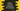# Python program to find the area and perimeter of a rectangle## Introduction :

If you know the formula, you can write a program to do that easily. In this tutorial, I will show you how to find the area and perimeter of a rectangle using user inputs. Our program will take the inputs from the user and print out the final results.

Before moving to the actual program, let me quickly show you the formula to find out the rectangle area, perimeter.

### Formula :

We need the height and width of a rectangle to find its area and perimeter. Formula to find the area is :

``Area = height * width``

And the formula to find the perimeter is :

``Perimeter = 2 * (height + width)``

The area is the amount of space it is covering and the perimeter is the total distance around its edges.

So, our program will ask the height and width from the user and calculate its area and perimeter using the above formulae. That’s it. Now let’s dive into the python program.

## Python program :

The below program will get the height and width from the user and prints out the area and perimeter. If you are familiar with the basics of python, it will not take a lot of time to understand the program. If you can’t understand it, go through the step by step explanation below.

``````# 1
width = float(input("Enter the width : "))
height = float(input("Enter the height : "))

# 2
area = width * height
perimeter = 2 * (width + height)

# 3
print("Area : {0:.2f}".format(area))
print("Perimeter : {0:.2f}".format(perimeter))``````

### Explanation :

The commented numbers in the above program denote the step numbers below :

1. We are reading the user inputs as float. Because the width and height could be anything like 12.3, 13.45, etc. right? The width of the rectangle is stored in the variable width and height is stored in the variable height.
2. Calculating the area and perimeter is straight forward. The variable area is used to store the area and perimeter is used to store the perimeter.
3. Print the area and perimeter on the console. Both area and perimeter are floats because width and height are floats. {0:.2f} is used to print the float value up to two decimal places.

### Sample Outputs :

``````Enter the width : 100
Enter the height : 10
Area : 1000.00
Perimeter : 220.00

Enter the width : 12.44
Enter the height : 23.45
Area : 291.72
Perimeter : 71.78

Enter the width : 33.45
Enter the height : 545.5
Area : 18246.98
Perimeter : 1157.90``````